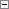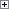Calculates the absolute value of a number (Decimal, Integer or BigInteger). ABS always returns a positive numeric value or zero.

NewNumber := ABS(Number)

Number

Type: Integer

The input value.Property Value/Return Value

Type: Integer

The return value is greater than or equal to 0 (zero).Remarks

The system automatically converts all of the numeric data types for you.Example

This example shows how to remove the sign from a negative numeric value. This example requires that you create the following variables and text constant in the C/AL Globals window.

Variable name DataType

x

Decimal

y

Decimal

Text constant name ENU Value

Text000

x = %1, y = %2Copy Code
x := -10.235; // x is assigned a negative value
y := ABS(x); // y is assigned the value of x without sign
MESSAGE(Text000, x, y);

The message window displays the following:

x = -10.235, y = 10.235